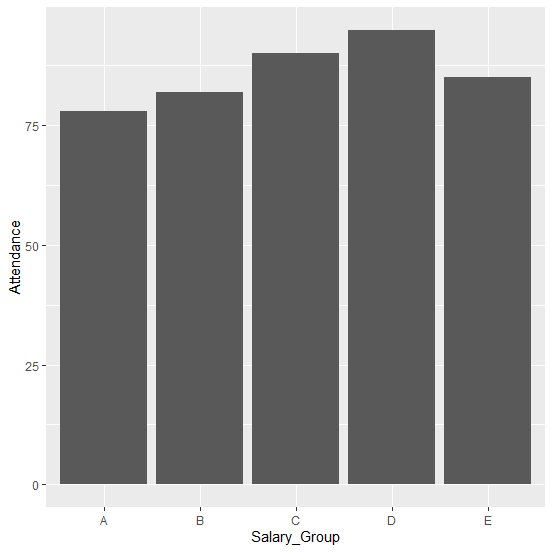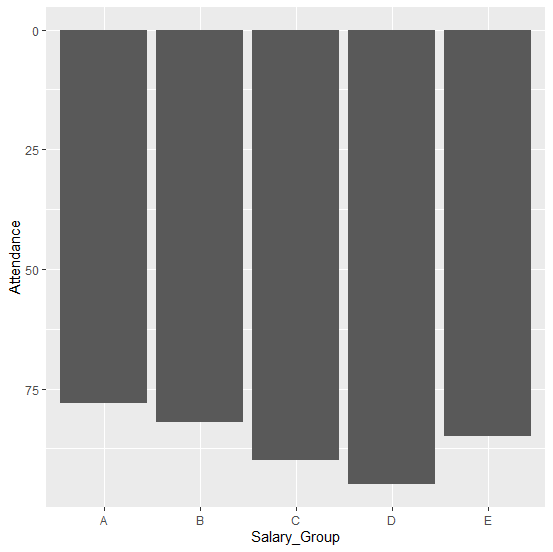# How to reverse the bars of a bar plot a using ggplot2 in R?

The bars of a bar plot are generally vertical from bottom to top but we can reverse them as well. Although, this is not a normal practice but we can do it if we want to. For this purpose, we will have to reverse the values on the Y-axis, as a result the bars will be reversed. It can be achieved by using scale_y_continuous.

## Example

Consider the below data frame −

Salary_Group <-c("A","B","C","D","E")
Attendance <-c(78,82,90,95,85)
df <-data.frame(Salary_Group,Attendance)
df

## Output

Salary_Group Attendance
1 A 78
2 B 82
3 C 90
4 D 95
5 E 85

Creating a simple bar plot −

## Example

library(ggplot2)
ggplot(df,aes(Salary_Group,Attendance))+geom_bar(stat="identity")

## OutputReversing the bars of the plot −

## Example

ggplot(df,aes(Salary_Group,Attendance))+geom_bar(stat="identity")+scale_y_continuous(trans="reverse")

## Output